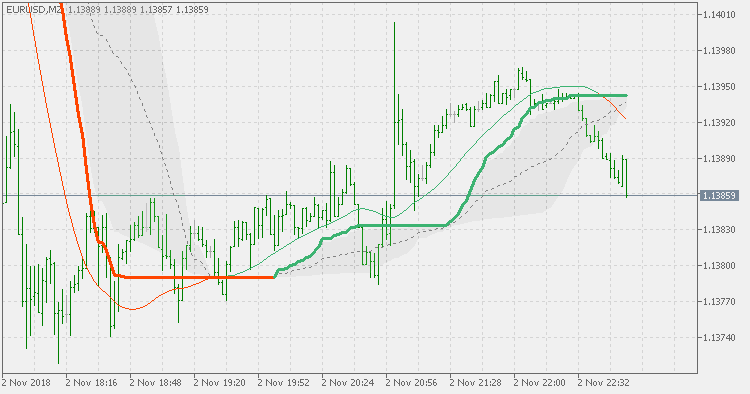Interesting script?
So post a link to it -
let others appraise it

You liked the script? Try it in the MetaTrader 5 terminal# Corrected generalized double DEMA - indicator for MetaTrader 5

Views:
2101
Rating:
Published:
2018.11.04 16:35
Updated:
2019.01.29 16:06

Theory:

The double exponential moving average (DEMA), was developed by Patrick Mulloy in an attempt to reduce the amount of lag time found in traditional moving averages. It was first introduced in the February 1994 issue of the magazine Technical Analysis of Stocks & Commodities in Mulloy's article "Smoothing Data with Faster Moving Averages.". The way to calculate is the following:

The DEMA (Double Exponential Moving Average) calculations are based combinations of a single EMA and double EMA into a new EMA:
1. Calculate EMA
2. Calculate Smoothed EMA by applying EMA with the same period to the EMA calculated in the first step
3. Calculate DEMA
DEMA = 2 * EMA - 1 * (Smoothed EMA)

"Generalized" DEMA was developed further by replacing the 2* and 1* parts with "volume factors" and thus adjusting the "speed" of the result and double DEMA is calculated as GDEMA of GDEMA. It is an intermediate step between "simple GDEMA and T3 and, as such, is smoother than GDEMA, but less smooth than T3.

This version:

Is using the generalized double DEMA and is applying the "correcting" method invented by dr. Alexander Uhl to produce a new "corrected" generalized DEMA. It also has floating levels for easier trend determination. Coloring methods supported are the following:

• color change on slope change
• color change on outer (floating) levels cross
• color change on middle (floating) level cross
• color change on average (GDEMA) value cross

Usage:

Depending on the choice of color change, you can use the color change as signal.Corrected generalized DEMA

Corrected generalized DEMAWeis Waves

Volume Wave indicator originally idealized by Richard D. Wyckoff.Volume weighted moving average

Volume weighted moving averageCorrected volume weighted moving average

Corrected volume weighted moving average